# The BRDF

The BRDF is one of the most powerful mathematical paradigms for computing real-world lighting (see Figure 17.8). It is a mathematical function that describes how much light is reflected off surfaces when a light beam makes contact with those surfaces. We will begin by analyzing its parameters, so we can give a complete formulation of the function. To begin with, reflectance depends on the light position and viewer position. As we saw in our simple example at the beginning of the chapter (which is nothing but a special case BRDF), the light position affected the diffuse component as well as the specular component, and the viewer's position affected the specular component.

##### Figure 17.8. Teapot lit using a metallic-look BRDF function.In our equations, we will represent the light's position using two parameters, qi and fi, which represent the incoming light direction in spherical coordinates. View direction will be represented by qo, fo, which are in spherical coordinates as well and depict the outgoing view direction. Additionally, BRDF is a function of the wavelength of light. We saw this in our photon explanation of light transport mechanisms. Thus, we shall add a parameter called l, which depends on the wavelength.

In addition, light interacts differently with different areas of a surface because of its inner details and structure. A tiled floor can have different reflectances for different types of tiles, and a stone can have varying properties depending on the exact mix of its constituents. This characteristic of surfaces, called positional variance, is expressed in terms of two parameters, u and v. These parameters represent the position parameterized to texture space, which in the end is where materials are specified. So, combining all this, we get a general BRDF formulation in the form:

` BRDFl (qI, I, qo, o, u, v) `

Now, this is a very ambitious approach, and BRDFs are complex to compute. So, some simplifications are applied. For example, position dependency is usually skipped, so the BRDF is computed per material. Such a BRDF is said to be position-invariant or shift-invariant, and is expressed by:

` BRDFl (qI, fI, qo, fo) `

We have now seen how the BRDF depends on angles that are usually expressed in terms of spherical coordinates. These coordinates are continuous values representing a unit sphere. But for computation purposes, we will discretize the interval and define the notion of differential solid angles as the area of a small rectangular region in the unit sphere. It is a powerful concept because it allows us to represent light in terms of energy flow through an area, which is expressed in Watts/m2. The units for solid angles are steradians.

Let's try to shed some light on the value of a BRDF. For an incoming light direction wi (a differential solid angle) and a viewer direction wo (solid angle as well), the BRDF has a value related to Lo (the amount of light reflected) and Ei (the amount of light arrived at the surface). The ratio is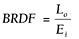Now, let's analyze the denominator. Ei represents the amount of light that arrives at the surface from direction wi. So how does that relate to the actual light intensity emitted from the original light source? Well, assuming Li is the emitted intensity, we must understand that we need to modulate the light intensity with regard to the incident angle, because the incoming light intensity must be projected onto the surface element (vertical light produces more intensity than sideways light, and so on). This projection is similar to the projection that happens with diffuse lighting as explained at the beginning of the chapter and can be simulated by modulating that amount by cosqi = Nwi. This means Ei = Licosqidwi. As a result, a BRDF is given by the following computation: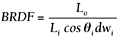And, after a couple pages of algebra, the global BRDF lighting equation is defined as: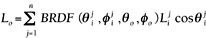with j denoting each light source's contribution. Several lighting models have been devised in terms of their BRDFs, and the Phong equation from earlier in this chapter is just one of them. The Torrance-Sparrow-Cook model, for example, is good for primarily specular surfaces. Other models are designed for rough diffuse surfaces. This is the case in the Oren-Nayar model and the Hapke/Lommel-Seeliger model, which were designed to model the dusty surface of the moon. Other interesting approaches are Minnaert, and on the complex end of the spectrum, He-Torrance-Sillion-Greenberg and Lafortune's Generalized Cosine Lobes, which both try to account for most observed phenomena.

That's all we need to know for now about BRDFs. We will now focus on how to code a BRDF into a real-time application. Remember that BRDFs are four-dimensional functions, and thus storing them into a 4D texture is out of question because of memory restrictions. Some alternative methods have been proposed and implemented successfully. The most popular one decomposes the 4D function as the multiplication of two 2D functions. Then, each 2D function is represented in a cube map (see Chapter 18), and multitexturing is used to perform the function multiply on the hardware. The tricky part is not the rendering. Cube maps have been around for quite a while now, and multitexturing is even older. The separation process is, on the other hand, pretty complex.

The process (called normalized decomposition) basically consists of the following separation:

` BRDF(qi, fi,qo, fo)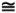G(qi, fi)·H(qo, fo). `

where G and H are the functions we will represent as cube maps. To compute them, we start by realizing that our BRDF could be computed (assuming we were to compute the 4D function) with code like this:

` double deltat = (0.5 * M_PI) / (16-1); double deltap = (2.0 * M_PI) / 16; double theta_i, phi_i; double theta_o, phi_o; for ( int h = 0; h < 16; h++ ) for ( int i = 0; i < 16; i++ ) for ( int j = 0; j < 16; j++ ) for ( int k = 0; k < 16; k++ )    {    theta_o  = h * deltat;    phi_o    = i * deltap;    theta_i  = j * deltat;    phi_i    = k * deltap; /* Compute or lookup the brdf value. */    val  = f( theta_i, phi_i, theta_o, phi_o )    /* Store it in a 4D array. */    BRDF[h][i][j][k] = val;    } `

This code assumes each value is sampled 16 times. Then, h represents qo, i represents fo, j represents qi, and k represents fi. We need to map this matrix to 2D. To do so, we unroll the 4D matrix into all combinations of parameter pairs. Each row and each column has two parameters fixed, and the other two varying in all possible combinations. The result is a larger matrix; each direction (rows and columns) being NxN in size. An example of such a matrix is shown here, where each parameter (theta and phi) has only two values, 0 and 1: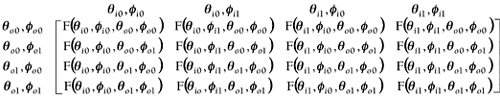If you understand the previous explanation of matrix unrolling, the following source code should be pretty easy to understand. Notice how we reindex the matrix positions to keep them mapped in 2D:

` double deltat = (0.5 * M_PI) / (N-1); double deltap = (2.0 * M_PI) / N; double theta_i, phi_i; double theta_o, phi_o; for ( int h = 0; h < N; h++ )  for ( int i = 0; i < N; i++ )   for ( int j = 0; j < N; j++ )    for ( int k = 0; k < N; k++ )     {     theta_o  = h * deltat;     phi_o    = i * deltap;     theta_i  = j * deltat;     phi_i    = k * deltap;     /* Compute or lookup the brdf value. */     val  = f( theta_i, phi_i, theta_o, phi_o );     /* Store it in a N2 x N2 matrix. */     BRDFMatrix[h*N+i][j*N+k] = val;     } `

It looks like this is getting more and more complex, so let's recapitulate for a second and regain perspective. We started with a 4D BRDF, which I assume we have at hand and can evaluate at any given moment. BRDF data is available from a number of sources. It can be a mathematical expression, tabulated data acquired through a goniometer, and so on. Then, all we do is map this 4D data set to a 2D matrix by using linear combinations of parameters. Why? Because this makes it easier to separate it afterward.

So, we will now perform the last step, which is to compute the separation based in this 2D representation. To do so, a two phase approach should be followed:

1. For each row, compute the norm of all BRDF values in that row.

2. For each column, divide each column value by its corresponding row-norm and compute the mean average of these "normalized" values.

Our 2D matrix is now full of numeric values. The norm we are talking about is the generalization of the Euclidean norm we are familiar with. Euclidean norm, also called the 2-norm, is defined by:

` (|x1|2 + |x2|2 + ... + |xn|2)1/2 `

The norm we will be using here is defined as:

` (|x1|n + |x2|n + ... + |xn|n)1/n `

This means we take each full row of the 2D matrix and compute its norm, storing all the results in a column vector N of positions. Let's call this vector of norms nvec. We then compute a new vector based on the columns. We scan each column and compute the difference in value between the matrix value and the norm corresponding to that column. Then, we store the average of these norm-matrix differences in a second vector, as shown here: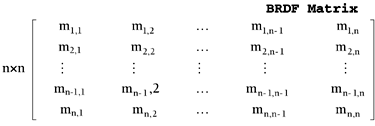#### Average Vector

The average vector must be computed per color component. Now, let's identify G and H. If you take a look at the row vector or norm vector, you will see that, due to the unrolling process, each value represents a sampled outgoing direction (qo,fo), which were the two parameters we fixed per row. So, this norm vector (identifying theta and phi as two parameters, and thus returning it to 2D form) is the function H(qo, fo). The average vector or column vector has a similar property: Each column is correlated to an incoming direction, so for each sampled incoming direction (qi,fi) there is a corresponding average value in the average vector. As a result, this average vector can serve as the function G(qi, fi).

As a result of this process, we can store two textures, G and H, which are the decomposition of the BRDF. By multiplying them, the whole BRDF matrix is generated. Remember that these textures (the matrices we have been talking about) are not indexed by position, but by the polar coordinates of the incoming and outgoing light direction. Theta and phi serve that purpose. All we need to do is create a cube map based on this angular shading data. By rendering it with multitexturing and a combine/multiply flag, we can effectively paint implement the BRDF.

The lighting models we have discussed so far, both the Phong and BRDF equations, account for scene lighting quite well. But they assume no occlusions ever take place, so shadows are not integrated into the equation. Rendering shadows in real time is a complex problem, and only recent advances in graphics hardware have allowed for general-purpose, robust techniques to appear. In this section, we will explore some of them.

A shadow is a dark region on a surface caused by the occlusion of incident light by another object (the occluder). Shadow extends along a prism with its apex at the light source and its edges along the silhouette of the occluder. In other words, a surface element is in shadow if (and only if) a ray from the element to the light source collides with the occluder prior to reaching the light.

Shadows are a per-pixel phenomena and are thus hard to compute. For some years, only special-case solutions were possible: shadows on a plane, shadows on very simple geometry, and so on. Today, at least two general-purpose algorithms can solve the issue properly.

Shadow mapping works by representing shadows by means of a shadow map, a special-case texture that represents the light and shadow information in a scene. The algorithm contains four steps:

1. Render the scene from the light's point of view and direction.

2. Use the light's depth buffer as a texture (shadow map).

3. Projectively texture the shadow map onto the scene.

4. Use "texture color" (comparison result) in fragment shading.

We start by setting the camera at the light source and aiming in the direction we want to compute shadows for. Then, we render the scene and read back the contents of the Z-buffer, which will become our shadow map. We do not need color, texturing, or lighting information, so all these features can be switched off to ensure that the shadow map is computed quickly. After we have created a texture out of the Z-buffer data, we need to use the shadow map information to render shadows to the scene. To do so, we render the scene again, this time setting the viewpoint at the camera location. Then, we need to merge the color information from this rendering pass with the shadowing information. This is the complex part of the algorithm, because the projection in both cases was computed from different orientations.

To solve this discrepancy in orientations, we use the approach described by Heidrich in his doctoral dissertation, subdividing the second step in two rendering passes. We first render the whole scene from the camera's viewpoint, with all lighting turned off and depth testing enabled. This paints the whole scene as if everything was in shadows. Then, a second pass and some clever alpha testing paints the lit areas, discarding shadowed fragments with the alpha test. The result is the combination of lit and unlit areas we expect. Take a look at Figure 17.9, where you can see both the result and the shadow map used to achieve it.

##### Figure 17.9. Left: resulting image. Right: shadow map that generates it.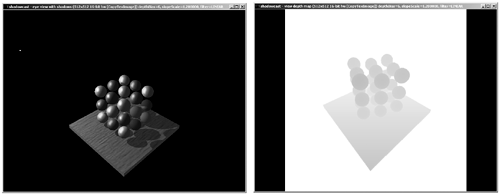In the second pass, we use two-unit multitexturing and register combiners or fragment shaders to implement the alpha testing. We assign the first texture unit to the depth map, which is stored in intensity format (RGBA with all channels storing the same value). The second texture unit is just a 1D linear ramp texture, which we will use to map the Z-values (from the viewpoint) to the [0..1] range. This way we can compare two textures based on their contents and actually perform a Z-comparison.

Obviously, we will need to create some specific projections, because the shadow map was initially created from the light's position. To create this projection matrix, we will rely on automatic texture coordinate generation functions, such as glTexGen.

For the first stage, which handles the shadow map, we must set the S, T, and Q texturing coordinates as follows:

` S        |1/2   0     0  1/2| T   =    |0    1/2    0  1/2|   * light projection * light modelview R        |0    0     0  0| Q        |0    0     0  1| `

Note how the R coordinate is not actually required to perform a 2D texture mapping. For the second stage, a similar approach will be used, but because the texture is 1D, only S and Q will be used. The matrix to pass to glTexGen is

` S        | 0 0 1 0 |    |1/2 0 0   1/2| T   =    | 0 0 0 0 | *  |0 1/2 0   1/2|  * light proj * light view R        | 0 0 0 0 |    |0  0 0   0 | Q        | 0 0 0 1 |    |0  0 0   1 | `

Then, setting TexGen is straightforward once we have the matrices. Take the S coordinate, for example. To set it from the resulting matrix, all we have to do is something like this:

` float p; p = m1; p = m1; p = m1; p = m1; glTexGenfv(GL_S, GL_EYE_PLANE, p); `

Now we have the matrices and texture maps. All we need to discuss is how we combine them to generate the alpha values. These values will accept or reject fragments depending on their depth. Remember that texture unit 0 holds the depth map, which is stored in all RGB and A channels. Texture unit 1 holds a 1D identity texture. But as we render the vertices, we will assign them texture coordinates based on their Z, so in the end we have two textures that have Z-values in their alpha channel. Thus, we only need to subtract these alphas (Z-values) and bias to 0.5. Thus, this operation assigns a resulting alpha value depending on the Z-comparison, so we can use this new alpha to reject or accept fragments depending on their relative depth. Specifically, fragments with Z-value (seen from the shadow map) larger than the Z-value seen from the viewpoint will be assigned alpha values larger than 0.5.

These fragments will be rejected, and vice versa. Here is the equation we are trying to implement:

` fragment alpha= alpha(Tex0) + (1 - alpha(Tex1)) - 0.5 `

For completeness, here is the full texture combiner source code needed to perform the required texture arithmetic for this operation:

` // activate the 0th texture unit glActiveTextureARB(GL_TEXTURE0_ARB); // combine textures: ON glTexEnvi(GL_TEXTURE_ENV, GL_TEXTURE_ENV_MODE, GL_COMBINE_EXT); // we want to replace the RGB value glTexEnvi(GL_TEXTURE_ENV, GL_COMBINE_RGB_EXT, GL_REPLACE); glTexEnvi(GL_TEXTURE_ENV, GL_SOURCE0_RGB_EXT, GL_PRIMARY_COLOR_EXT); // by the color of the source fragment glTexEnvi(GL_TEXTURE_ENV, GL_OPERAND0_RGB_EXT, GL_SRC_COLOR); // store the texture alpha as well glTexEnvi(GL_TEXTURE_ENV, GL_COMBINE_ALPHA_EXT, GL_REPLACE); glTexEnvi(GL_TEXTURE_ENV, GL_SOURCE0_ALPHA_EXT, GL_TEXTURE); glTexEnvi(GL_TEXTURE_ENV, GL_OPERAND0_ALPHA_EXT, GL_SRC_ALPHA); // activate the 1th texture unit glActiveTextureARB(GL_TEXTURE1_ARB); glTexEnvi(GL_TEXTURE_ENV, GL_TEXTURE_ENV_MODE, GL_COMBINE_EXT); glTexEnvi(GL_TEXTURE_ENV, GL_COMBINE_RGB_EXT, GL_REPLACE); // take the color from the previous stage glTexEnvi(GL_TEXTURE_ENV, GL_SOURCE0_RGB_EXT, GL_PREVIOUS_EXT); glTexEnvi(GL_TEXTURE_ENV, GL_OPERAND0_RGB_EXT, GL_SRC_COLOR); // add signed implements the interpolation function glTexEnvi(GL_TEXTURE_ENV, GL_COMBINE_ALPHA_EXT, GL_ADD_SIGNED_EXT); glTexEnvi(GL_TEXTURE_ENV, GL_SOURCE0_ALPHA_EXT, GL_PREVIOUS_EXT); glTexEnvi(GL_TEXTURE_ENV, GL_OPERAND0_ALPHA_EXT, GL_SRC_ALPHA); glTexEnvi(GL_TEXTURE_ENV, GL_SOURCE1_ALPHA_EXT, GL_TEXTURE); // filtered by the alpha glTexEnvi(GL_TEXTURE_ENV, GL_OPERAND1_ALPHA_EXT, GL_ONE_MINUS_SRC_ALPHA); `

And here is the DirectX equivalent to the preceding code:

` // Set the base texture. d3dDevice->SetTexture(0,lptexBaseTexture ); // Set the base texture operation and args. d3dDevice->SetTextureStageState(0,D3DTSS_COLOROP, D3DTOP_SELECTARG1); // first operator: the base texture d3dDevice->SetTextureStageState(0,D3DTSS_COLORARG1, D3DTA_TEXTURE ); // Set the interpolated texture on top. d3dDevice->SetTexture(1,lptexSecondTexture); // Set the blend stage. We want to do a signed add d3dDevice->SetTextureStageState(1, D3DTSS_COLOROP, D3DTOP_ADDSIGNED); // first parameter is the light map d3dDevice->SetTextureStageState(1, D3DTSS_COLORARG1, D3DTA_TEXTURE ); // second parameter is the previous stage d3dDevice->SetTextureStageState(1, D3DTSS_COLORARG2, D3DTA_CURRENT ); `

After this pass has been performed and the scene rendered, the results are as follows:

• RGB is lit color (lighting is enabled during second pass).

• Alpha is the biased difference between both textures.

• Unshadowed fragments have an alpha > = 0.5.

• Shadowed fragments have an alpha < = 0.5.

So all we have to do is enable alpha testing with a threshold of 0.5 and we're all set:

` glEnable(GL_ALPHA_TEST); glAlphaTest(GL_GREATER, 0.5); `

A couple words of advice about the preceding technique: First, shadow mapping largely depends on the size of the shadow maps. Being a texture-based algorithm, the larger the shadow map, the smoother the shadows. Some games show staircase patterns in their shadows due to shadow maps being too small. On the other hand, this can become a memory issue.

Second, a more subtle piece of advice: Sometimes, your depth map viewpoint comparisons will generate inaccurate results because of the finite resolution of the shadow map and the Z-buffer. On one hand, remember that our shadow map is 8-bits only. To handle this, I recommend you take a look at the higher color depth textures available under modern hardware. On the other hand, even with the highest resolution, sometimes fragments that are supposed to be lit will appear shadowed. The reason for this is very simple: As you render the shadow map, you are effectively storing the Z-values (up to the Z-buffer resolution) at which the first object was found. Then, in the second pass, when you render the same pixel, minimal Z-buffer differences can cause the fragment to be caught in its own shadow. If the Z-value of the second pass is slightly beyond what was computed on the first pass, the sphere's surface will be "inside" the sphere's surface, and we will consider it shadowed. The solution to this problem is easy. Use calls like glPolygonOffset to shift Z-values slightly in the shadow map computation pass so the stored Z-values are a bit on the conservative side. By doing so, we can ensure that a surface will always be "outside" of its shadow.

Shadow mapping quality is a result of shadows based on a texture mapping approach. But we can approach shadows from a wholly different perspective. Imagine that you take the light source and construct a frustum that passes through the light source and through all vertices in the silhouette of the object acting as the occluder. If you examine the shape of the frustum, you will realize it's a volume that designates the area in space covered by shadows. Somehow, we could try to render shadows not based on texture maps, but on the shadow volume they project. This way our solution would be resolution independent: no jaggies, just perfectly straight lines. This approach is implemented via a special hardware device called the stencil buffer. So the technique we will now cover is called stencil shadows.

Stencil shadows work by somehow "painting" the shadow volume and using it as information for the renderer. To use this information, the renderer takes advantage of the stencil buffer. So before moving on, it would be a good idea to review what the stencil shadow is and what it can do for us. The stencil buffer is an offscreen buffer that we can select and paint into. The peculiarity is that whenever we paint into the stencil buffer, we create a mask. The shapes we paint there are stored, and then afterward, we can render in "normal" mode and paint only if the stencil buffer is set to a specific value. The first use of the stencil buffer was as a clipping region delimiter. Imagine that you need to render through a strange-shaped window. You would enable the stencil buffer, render the shape of the window, and then render whatever you actually wanted to paint, with stencil testing enabled.

So how can a stencil buffer help us paint realistic shadows? Let's first review the abstract algorithm and later implement it using the stencil buffer. The idea is simple: On the first pass, we render the shadow volume with culling disabled, so both front-and back-facing primitives are rendered. Then, on a per-pixel basis, we shoot a ray from the viewpoint and through the pixel being shaded. If the pixel does eventually reach an object, we must count the number of collisions between the ray and the sides of the shadow volume. Each front-facing intersection adds one to a counter, whereas each back-facing intersection decrements the counter by one. The object is shadowed if the counter retains a positive value before we reach it. Unshadowed objects are defined by counter values equal to zero. Take a look at Figure 17.10, where the shadow volume used for initializing the stencil is clearly shown.Let's now implement the previous algorithm, taking advantage of the stencil buffer. Here is the outline:

` clear the frame buffer render the visible scene using ambient light (this renders everything in shadows) clear the stencil buffer for each light source,    determine objects that may cast shadows in the visible region of the world.    for each object       calculate the silhouette from the light source       extrude the silhouette from the light to form a shadow volume       enable writing to the stencil buffer       render front part of volume, incrementing stencil on z-pass       render back part of volume, decrementing stencil on z-pass    end for    enable reading from the stencil buffer    render the scene with lighting on, painting where the stencil is zero end for `

The stencil buffer actually counts intersections for us, and we are allowed to read its contents back. Let's refine some of the main portions of the algorithm so the implementation details become obvious. We first need to render the scene with lights off, so everything is in shadow. Then, we clear the stencil so we can begin pouring the geometry and shadow volume data. In this first code snippet, we clear the stencil buffer, enable stenciling so it always passes, and set the depth test and function:

` glClear(GL_STENCIL_BUFFER_BIT); glEnable(GL_STENCIL_TEST); glStencilFunc(GL_ALWAYS, 0, 0); glEnable(GL_DEPTH_TEST); glDepthFunc(GL_LESS); `

We are only going to draw into the stencil buffer, so we need to disable writes to the color buffer and depth buffer as follows in order to guarantee maximum efficiency:

` glColorMask(GL_FALSE, GL_FALSE, GL_FALSE, GL_FALSE); glDepthMask(GL_FALSE); `

We have reached the point when we have to actually render the shadow volume, increasing or decreasing the intersection count as needed. This might seem complex, but can be achieved with these simple lines of code:

` glEnable(GL_CULL_FACE); glCullFace(GL_BACK); glStencilOp(GL_KEEP, GL_KEEP, GL_INCR); DrawShadowVolume(); glCullFace(GL_FRONT); glStencilOp(GL_KEEP, GL_KEEP, GL_DECR); DrawShadowVolume(); `

We then render the lighting pass, which effectively adds illumination to the scene. This is achieved using the following code:

` glColorMask(GL_TRUE, GL_TRUE, GL_TRUE, GL_TRUE); glDepthFunc(GL_EQUAL); glStencilFunc(GL_EQUAL, 0, 0); glStencilOp(GL_KEEP, GL_KEEP, GL_KEEP); glEnable(GL_BLEND); glBlendFunc(GL_ONE, GL_ONE); `

The same sequence can be mapped easily to DirectX. DirectX code tends to be a bit longer sometimes, so make sure you refer to the preceding OpenGL sample if you get lost. That said, here is the step-by-step code, rewritten for DirectX 9. We clear the stencil buffer, enable stenciling so it always passes, and set the depth test and function:

` d3dDevice->SetRenderState(D3DRS_STENCILENABLE, TRUE); d3dDevice->SetRenderState(D3DRS_STENCILFUNC,D3DCMP_ALWAYS); d3dDevice->SetRenderState(D3DRS_ZENABLE, TRUE); d3dDevice->SetRenderState(D3DRS_ZFUNC,D3DCMP_LESS); `

To write to the stencil buffer we disable color and Z-writing for maximum efficiency, using the lines:

` d3dDevice->SetRenderState(D3DRS_COLORWRITEENABLE, FALSE); d3dDevice->SetRenderState(D3DRS_ZWRITEENABLE, FALSE); `

Again, we have reached the point when our DirectX code needs to actually render the shadow volume, increasing or decreasing the intersection count as needed. Here is the DirectX version of the code:

` d3dDevice->SetRenderState(D3DRS_CULLMODE, D3DCULL_CW); d3dDevice->SetRenderState(D3DRS_STENCILFAIL, D3DSTENCILCAPS_KEEP); d3dDevice->SetRenderState(D3DRS_STENCILZFAIL, D3DSTENCILCAPS_KEEP); d3dDevice->SetRenderState(D3DRS_STENCILPASS, D3DSTENCILCAPS_INCR); DrawShadowVolume(); d3dDevice->SetRenderState(D3DRS_CULLMODE, D3DCULL_CCW); d3dDevice->SetRenderState(D3DRS_STENCILFAIL, D3DSTENCILCAPS_KEEP); d3dDevice->SetRenderState(D3DRS_STENCILZFAIL, D3DSTENCILCAPS_KEEP); d3dDevice->SetRenderState(D3DRS_STENCILPASS, D3DSTENCILCAPS_DECR); DrawShadowVolume(); `

We then need to render the lighting pass, which effectively adds illumination to the scene. This is achieved using the following DirectX code:

` d3dDevice->SetRenderState(D3DRS_COLORWRITEENABLE, TRUE); d3dDevice->SetRenderState(D3DRS_ZFUNC,D3DCMP_EQUAL); d3dDevice->SetRenderState(D3DRS_STENCILFUNC, D3DCMP_EQUAL); d3dDevice->SetRenderState(D3DRS_STENCILREF, 0); d3dDevice->SetRenderState(D3DRS_STENCILMASK, 0); d3dDevice->SetRenderState(D3DRS_STENCILFAIL, D3DSTENCILCAPS_KEEP); d3dDevice->SetRenderState(D3DRS_STENCILZFAIL, D3DSTENCILCAPS_KEEP); d3dDevice->SetRenderState(D3DRS_STENCILPASS, D3DSTENCILCAPS_KEEP); d3dDevice->SetRenderState(D3DRS_ALPHABLENDENABLE, TRUE); d3dDevice->SetRenderState(D3DRS_SRCBLEND, D3DBLEND_ONE); d3dDevice->SetRenderState(D3DRS_DESTBLEND, D3DBLEND_ONE); `

Notice that we use a depthfunc of GL_LEQUAL, so we're effectively overwriting those fragments already on the Z-buffer.

As with shadow maps, stencil shadows have some issues we must learn to deal with. First and foremost, there's the silhouette extraction process. The classic way to compute it is to store edge connectivity information, so we can detect edges whose neighboring triangles face one in each direction with regard to the viewpoint. These are the edges that make up the silhouette. Currently, much work is being done in computing the extruded shadow volume in a vertex program. Whichever you choose, algorithmic cost is always going to be O(number of triangles). That's why many games and algorithms use lower quality meshes for the silhouette-extrusion process. We are very sensitive to the existence or absence of a shadow, but we are not extremely picky when it comes to its shape. Thus, using a lower resolution mesh will probably do the job equally well.

A second potential problem is handling camera in-shadow scenarios: What happens if our camera is inside the shadow volume? All our cleverly laid out stencil buffer math stops working because the crossings count does not mean what it's supposed to. The rule here is to render the shadow volume as well as the caps so we have a closed object and change the stencil tests to:

` glCullFace(GL_FRONT); glStencilOp(GL_KEEP, GL_INCR, GL_KEEP); DrawShadowVolume(); glCullFace(GL_BACK); glStencilOp(GL_KEEP, GL_DECR, GL_KEEP); DrawShadowVolume(); `

Or, if you prefer DirectX, to something like this:

` d3dDevice->SetRenderState(D3DRS_CULLMODE, D3DCULL_CW); d3dDevice->SetRenderState(D3DRS_STENCILFAIL, D3DSTENCILCAPS_KEEP); d3dDevice->SetRenderState(D3DRS_STENCILZFAIL, D3DSTENCILCAPS_INCR); d3dDevice->SetRenderState(D3DRS_STENCILPASS, D3DSTENCILCAPS_KEEP); DrawShadowVolume(); d3dDevice->SetRenderState(D3DRS_CULLMODE, D3DCULL_CCW); d3dDevice->SetRenderState(D3DRS_STENCILFAIL, D3DSTENCILCAPS_KEEP); d3dDevice->SetRenderState(D3DRS_STENCILZFAIL, D3DSTENCILCAPS_DECR); d3dDevice->SetRenderState(D3DRS_STENCILPASS, D3DSTENCILCAPS_DECR); DrawShadowVolume(); `

This changes the behavior, so when the depth test fails, we increase/decrease instead of doing so when the test passes. By doing so, we ensure that the rest of the algorithm works as expected. All we need to do is create the caps, which can be done in the same pass where we extrude the shadow volume.Core Techniques and Algorithms in Game Programming2003
ISBN: N/A
EAN: N/A
Year: 2004
Pages: 261